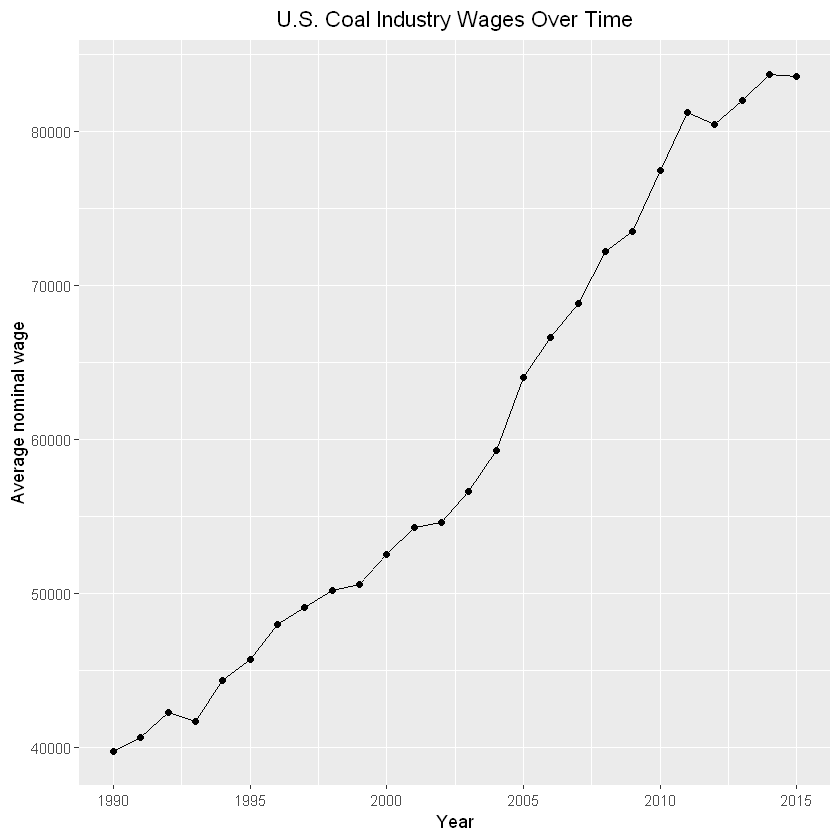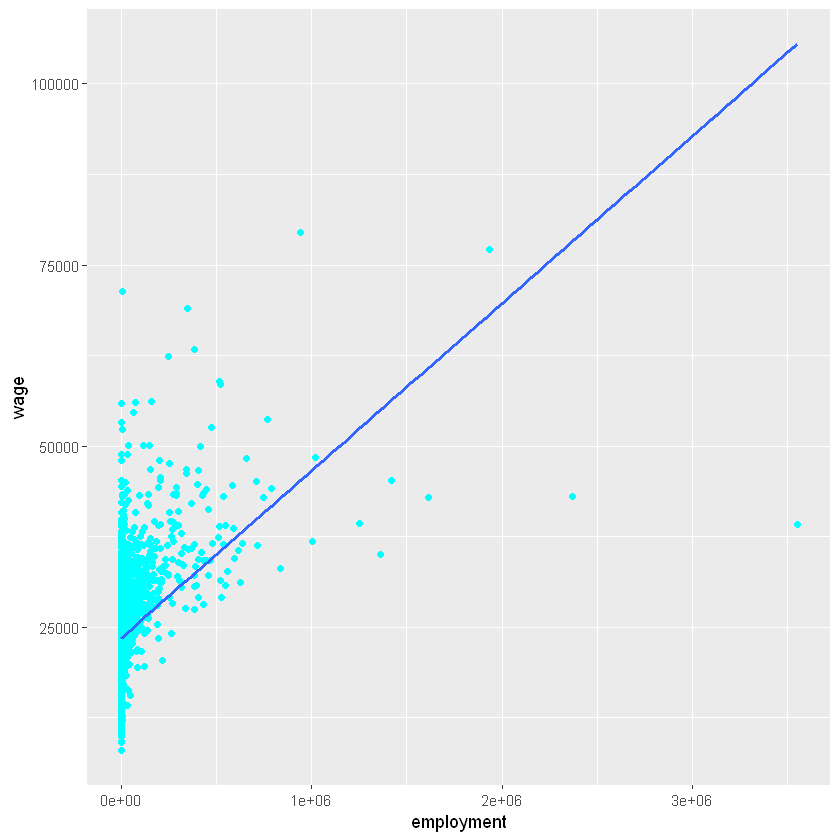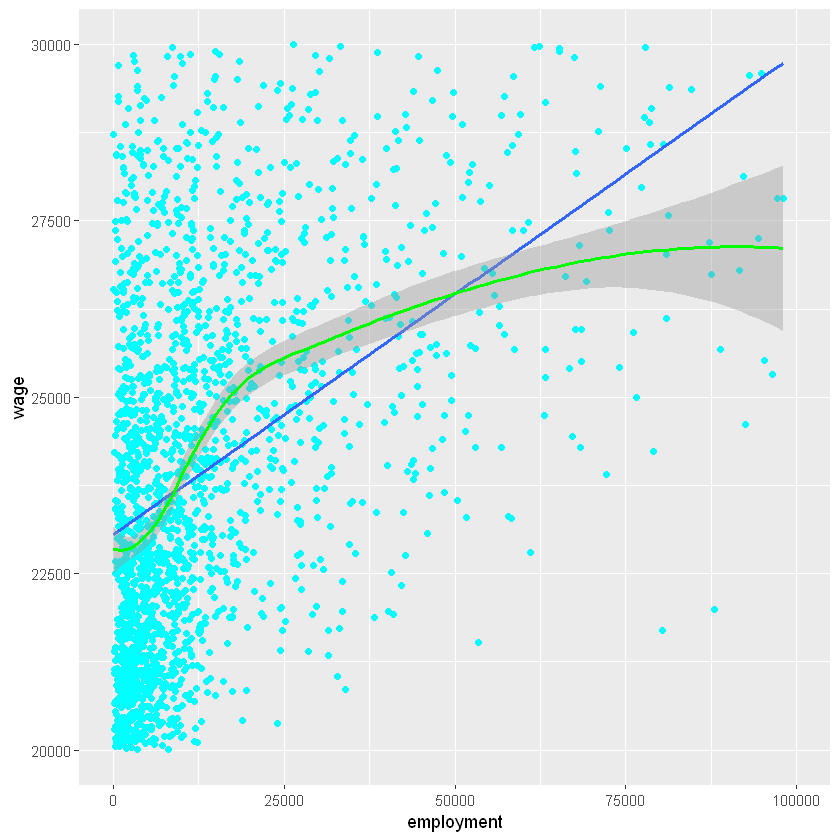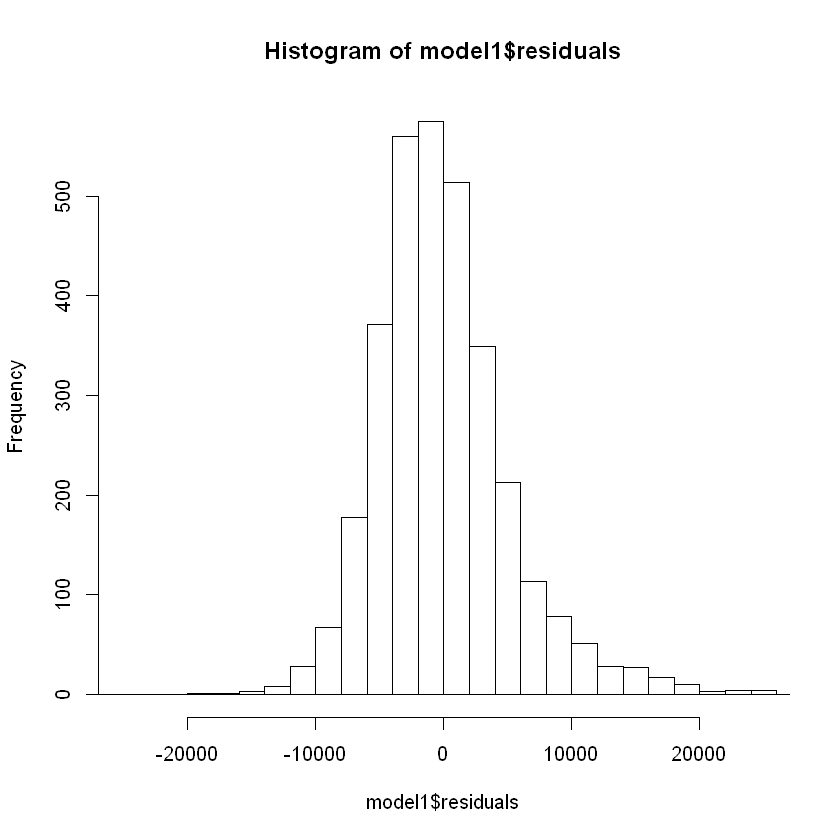# Variables, if statements, functions, printing output ¶

In :
x<-3
print(x)

 3

In :
if(x<5){
print("The value of x is less than 5")
} else{
print("The value of x is greater than or equal to 5")
}

 "The value of x is less than 5"

In :
add5<-function(x){
if(is.numeric(x)){
}
else{
print("Argument x is not a number")
return(0)
}
}
print(paste0("42 plus five is equal to: ", add5(52)))

 "42 plus five is equal to: 57"

In :
print(add5("sdafgdsfg"))

 "Argument x is not a number"
 0

In :
running_sum<-0
for (i in 1:10){
running_sum = running_sum+i
}
print(running_sum)

 55

In :
1:10

1. 1
2. 2
3. 3
4. 4
5. 5
6. 6
7. 7
8. 8
9. 9
10. 10

# Data frames ¶

### The basic useful way to manage data in R is with a so-called data frame. We can create one manually:¶

In :
df<-data.frame(variable1 = c(5,7,9), variable2=c(2,4,6))
df

variable1variable2
52
74
96

### It's easy to add functions of variables as new variables. For renaming and dropping variables, see the next lesson notebook.¶

In :
df$variable2_squared <- df$variable2^2
df

variable1variable2variable2_squared
5 2 4
7 4 16
9 6 36

## To make things more interesting, let's loading in some real data.¶

### This data gives the US average wage by industry and year, from the Quarterly Census of Employment and Wages¶

In :
projectpath<-"C:/Users/Len/Dropbox/Teaching/Data TA/R tutorial/sample project/"


## Exploring/working with a dataframe by "slicing" it, i.e. indexing it as a matrix¶

### We can look at the first 5 rows of the dataframe:¶

In :
df[1:5,c('naics','wage')]

naicswage
1111 14667.21
1112 12264.32
1113 10557.79
1114 14888.62
1119 13482.87
In :
# A "comment" in R can be made with the "#" symbol
# Let's ask R to print how many records are in df:
print(length(df[,1]))

 8008

In :
#First five values of wage only:
df$wage[1:5]  1. 14667.2116085861 2. 12264.3232139683 3. 10557.7870162356 4. 14888.6238310147 5. 13482.8713409426 In : print(paste0("Mean industry wage: ", mean(df$wage)))
print(paste0("Max industry wage: ", max(df$wage)))   "Mean industry wage: 41390.2396774684"  "Max industry wage: 226189.599662489"  In : #What if I want to display these more pretty-like? print(paste0("Mean industry wage:$", round(mean(df$wage),2))) print(paste0("Mean industry wage:$", round(mean(df$wage),-3)/1000, "k"))   "Mean industry wage:$41390.24"

# An example of plotting ¶

## Suppose we wanted to plot average nominal wages for the coal industry over time:¶

In :
#Step one: create a dataframe with average manufacturing wage, by year
dfcoal<-df[df$naics==2121,] dfcoal  yearnaicswage 211990 2121 39767.71 3301991 2121 40668.72 6391992 2121 42295.11 9481993 2121 41716.56 12571994 2121 44389.39 15661995 2121 45734.90 18751996 2121 48031.03 21841997 2121 49145.38 24931998 2121 50243.96 28021999 2121 50638.49 31112000 2121 52560.33 34202001 2121 54335.28 37302002 2121 54639.26 40402003 2121 56627.55 43502004 2121 59325.00 46602005 2121 64058.03 49702006 2121 66638.51 52802007 2121 68861.89 55862008 2121 72240.77 58922009 2121 73500.63 61982010 2121 77466.31 65042011 2121 81257.74 68092012 2121 80450.46 71142013 2121 82067.37 74192014 2121 83749.85 77242015 2121 83594.60 ## We'll use the ggplot plotting library for this, which must be installed. ggplot has a formula based syntax that takes some getting used to.¶ In : #install.packages("ggplot2") library(ggplot2) #ggplot titles by default show up on the left. I like them in the middle: theme_update(plot.title = element_text(hjust = 0.5)) ggplot(data=dfcoal, aes(x=year, y=wage, group=1)) + geom_line()+ geom_point()+ labs(title="U.S. Coal Industry Wages Over Time", x="Year", y = "Average nominal wage")## Follow up question ("extra credit"): have these nominal wages kept up with inflation? You could find out by integrating data from here .¶ ## We can also save this plot to a file (e.g. to put it in our paper). Remember the project folder!¶ In : ggsave(filename = paste0(projectpath,'figures/coal_wagesbyyear.png'), width = 10, height = 5, dpi=500)  # Logging output ¶ ## If you have experience with Stata, you might be used to creating a "log file" from your code. We can do a similar thing in R, using the sink command:¶ In : projectpath<-"C:/Users/Len/Dropbox/Teaching/Data TA/R tutorial/sample project/" #split=TRUE allows output to also go to the screen (otherwise you would only see it in the log file) #append=FALSE tells R to overwrite "mylog.txt" rather than appending to itself, if it already exists sink(file=paste0(projectpath,'/log files/mylog.txt'),split=TRUE, append=FALSE)  ## This doesn't work in a Jupyter notebook, so copy this code into R Studio¶ ### Note: it's good practice to close the connection to this file at the end of your code (this makes more sense when working in RStudio in which your have a ".R" script file). The following code will close any open file connections:¶ In : sink.reset <- function(){ for(i in seq_len(sink.number())){ sink(NULL) } }  # Part II¶ # Regression analysis ¶ ## Let's move on to regression analysis. For this, download this data set to your raw data/ folder. Or, you can read it right of the web (and save a local copy):¶ In : df<- read.csv("http://www.columbia.edu/~ltg2111/teaching/wages_bycounty_yr2000.csv") write.csv(df, paste0(projectpath,"raw data/wages_bycounty_yr2000.csv"))  ## This data set contains the average wage and employment count by US county (fips') in the year 2000:¶ In : df <- read.csv(paste0(projectpath,"raw data/wages_bycounty_yr2000.csv")) df[1:5,]  Xfipswageemployment 1 1001 24803.96 8844 2 1003 21926.4540999 3 1005 22849.07 9890 4 1007 20039.41 2686 5 1009 21934.78 7372 ## Suppose we want to run a regression of wages on employment in this data. If we just wanted to visualize the regression line, we could let ggplot do it all automatically:¶ In : #Scatterplot of wage vs employment with regression line library(ggplot2) ggplot(data = df, aes(x = employment,y = wage)) + geom_point(color='cyan') + geom_smooth(method = "lm", se = FALSE)### Note: the above relationship does not look particularly linear overall. We might want to add employment squared as a regresssor as well, or use so-called non-parametric regression techniques that let the curve take an arbitrary shape.¶ ### By comparing the linear regression line to the loess local smoother, we can assess the linearity if the relationship. Here we zoom in on counties with average wage between 20-30k and employment below 100k, where the bulk of the data is.¶ In : ggplot(data = df, aes(x = employment,y = wage)) + ylim(20000, 30000) + xlim(0,10^5) + geom_point(color='cyan') + geom_smooth(method = "lm", se = FALSE) + geom_smooth(method = "loess", color="green")## Now let's perform the linear regression using the "linear model" or lm command to see the estimated coefficients:¶ In : lm(wage~employment,data=df)  Call: lm(formula = wage ~ employment, data = df) Coefficients: (Intercept) employment 2.345e+04 2.311e-02  In : #It's usually useful to save the estimated model as an object: model1<-lm(wage~employment,data=df) summary(model1)  Call: lm(formula = wage ~ employment, data = df) Residuals: Min 1Q Median 3Q Max -66231 -3382 -681 2471 47744 Coefficients: Estimate Std. Error t value Pr(>|t|) (Intercept) 2.345e+04 1.041e+02 225.33 <2e-16 *** employment 2.311e-02 8.014e-04 28.84 <2e-16 *** --- Signif. codes: 0 '***' 0.001 '**' 0.01 '*' 0.05 '.' 0.1 ' ' 1 Residual standard error: 5701 on 3214 degrees of freedom Multiple R-squared: 0.2056, Adjusted R-squared: 0.2053 F-statistic: 831.7 on 1 and 3214 DF, p-value: < 2.2e-16  ## Important! The standard errors that lm computes by default assume homoskedasticity, which is not reasonable in most social science contexts. Based on the above scatterplot, would it be reasonable to assume homoskedastic errors for this regression? Doesn't look like it.¶ ## To get heteroskedasticity-robust standard errors, you can use the sandwich library: ¶ In : #install.packages("sandwich") library(sandwich) cov <- vcovHC(model1, type = "HC") model1.robustses <- sqrt(diag(cov)) model1.robustses  (Intercept) 171.42093890755 employment 0.00506838094830457 In : model1$coefficients

(Intercept)
23452.3821454205
employment
0.0231118203981946
In :
#If we wanted to supress the constant:
lm(wage~employment+0,data=df)

Call:
lm(formula = wage ~ employment + 0, data = df)

Coefficients:
employment
0.06989

In :
#Suppose we wanted to inspect the residuals:
hist(model1$residuals, breaks=50,xlim=c(-25000,25000))# Merging Data ¶ ## Now we've found another data source that includes unemployment by county in 2016. How can we combine this with our existing dataset, to perform a multiple linear regression?¶ ## This data is available here. Download it and save to your raw data/ folder.¶ In : temp<- read.csv("https://raw.githubusercontent.com/plotly/datasets/master/fips-unemp-16.csv") write.csv(temp, paste0(projectpath,"raw data/fips-unemp-16.csv"))  In : df2<-read.csv(paste0(projectpath,"raw data/fips-unemp-16.csv"))  ## We can perform the merge using the merge command:¶ In : merged_data <- merge(df, df2, by="fips", all.x = TRUE, all.y=FALSE) merged_data[1:5,]  fipsX.xwageemploymentX.yunemp 1001 1 24803.96 8844 1 5.3 1003 2 21926.4540999 2 5.4 1005 3 22849.07 9890 3 8.6 1007 4 20039.41 2686 4 6.6 1009 5 21934.78 7372 5 5.5 ### Note that by here can be a list of variables to merge on combinations of, e.g. by=c(var1, var2).¶ ### The options all.x = TRUE, all.y=FALSE makes sure to keep all of our original county observations, even if we can't find an unemployment number for them. Counties that show up only in the unemployment dataset are ignored.¶ ### We can see that 9 counties were unmatched, because they have NA's for unemp:¶ In : summary(merged_data) merged_data[is.na(merged_data$unemp),]

      fips            X.x              wage         employment
Min.   : 1001   Min.   :   1.0   Min.   : 8053   Min.   :     19
1st Qu.:19039   1st Qu.: 804.8   1st Qu.:20264   1st Qu.:   2265
Median :30028   Median :1608.5   Median :23167   Median :   6308
Mean   :31464   Mean   :1608.5   Mean   :24230   Mean   :  33644
3rd Qu.:46114   3rd Qu.:2412.2   3rd Qu.:26846   3rd Qu.:  18226
Max.   :78030   Max.   :3216.0   Max.   :79544   Max.   :3548191

X.y             unemp
Min.   :   1.0   Min.   : 1.700
1st Qu.: 809.5   1st Qu.: 4.000
Median :1611.0   Median : 5.000
Mean   :1612.3   Mean   : 5.456
3rd Qu.:2415.5   3rd Qu.: 6.300
Max.   :3219.0   Max.   :23.500
NA's   :9        NA's   :9       
fipsX.xwageemploymentX.yunemp
86 2201 86 27511.05 1165 NA NA
88 2232 88 28550.83 1068 NA NA
91 2270 91 16724.99 878 NA NA
92 2280 92 25470.78 1736 NA NA
241246113 2412 22689.80 2244 NA NA
290951515 2909 25314.70 3970 NA NA
321478010 3214 28077.1212053 NA NA
321578020 3215 20178.25 1991 NA NA
321678030 3216 22985.7114565 NA NA

### On the rest of the data, let's do an OLS regression of wage on employment count and unemployment rate (counties with missing unemp will be ignored by the lm command):¶

In :
model2<-lm(wage~employment+unemp,data=merged_data)
summary(model2)

Call:
lm(formula = wage ~ employment + unemp, data = merged_data)

Residuals:
Min     1Q Median     3Q    Max
-64582  -3441   -673   2457  48149

Coefficients:
Estimate Std. Error t value Pr(>|t|)
(Intercept)  2.565e+04  2.575e+02  99.590   <2e-16 ***
employment   2.261e-02  7.934e-04  28.501   <2e-16 ***
unemp       -3.997e+02  4.294e+01  -9.308   <2e-16 ***
---
Signif. codes:  0 '***' 0.001 '**' 0.01 '*' 0.05 '.' 0.1 ' ' 1

Residual standard error: 5631 on 3204 degrees of freedom
(9 observations deleted due to missingness)
Multiple R-squared:  0.2267,	Adjusted R-squared:  0.2262
F-statistic: 469.6 on 2 and 3204 DF,  p-value: < 2.2e-16

In :
# Again, if we are interested in statistical inference on our coefficients, we should generally use
# standard errors that are valid under heteroskedasticity:
cov <- vcovHC(model2, type = "HC")
model2.robustses <- sqrt(diag(cov))
model2.robustses

(Intercept)
359.606291243014
employment
0.00495834913777904
unemp
50.0528772565186

## Aside: deleting, renaming, ordering variables in a dataframe ¶

### First, let's drop these meaningless variables X.x and X.y. These come from the fact that there was a variable named "X" in both datasets before the merge, which simply counted the row number.¶

In :
head(merged_data)

fipsX.xwageemploymentX.yunemp
1001 1 24803.96 8844 1 5.3
1003 2 21926.4540999 2 5.4
1005 3 22849.07 9890 3 8.6
1007 4 20039.41 2686 4 6.6
1009 5 21934.78 7372 5 5.5
1011 6 20437.67 2774 6 7.2
In :
somevars <- names(merged_data) %in% c("X.x", "X.y")
cleaned_data <- merged_data[!somevars]

fipswageemploymentunemp
1001 24803.96 8844 5.3
1003 21926.4540999 5.4
1005 22849.07 9890 8.6
1007 20039.41 2686 6.6
1009 21934.78 7372 5.5
1011 20437.67 2774 7.2

### Some other data frame manipulation tasks that may be useful:¶

In :
#Create a new variable that groups employment into 4 quartiles
qnt <- quantile(cleaned_data$employment) cleaned_data$employment_quartile<-cut(cleaned_data$employment,unique(qnt),include.lowest=TRUE) #Sort the data frame by employment cleaned_data <- cleaned_data[order(cleaned_data$employment),]

#Re-order first few columns in data frame.
#In this exmaple, make "employment" and "employment_quartile" first and retain ordering of the rest
somecols <- c("employment", "employment_quartile");
cols <- c(somecols, names(cleaned_data)[-which(names(cleaned_data) %in% somecols)]);
cleaned_data <- cleaned_data[cols]

#rename the "fips" variable to "county"
colnames(cleaned_data)[which(colnames(cleaned_data) == 'fips')[]] <- "county"


employmentemployment_quartilecountywageunemp
202819 [19,2.26e+03]38087 13620.74 2.5
162932 [19,2.26e+03]30069 16411.38 4.8
173150 [19,2.26e+03]31165 15092.88 2.8
161361 [19,2.26e+03]30037 15563.97 4.4
165361 [19,2.26e+03]31005 13003.02 6.2
165461 [19,2.26e+03]31007 19724.15 3.8

### For example, with data.table, we can quickly extract the average employment across counties within each quartile of employment¶

In :
library(data.table)
dt = data.table(cleaned_data)
dt <- dt[, .(mean(employment)), keyby = .(employment_quartile)]
#the column with mean employment ends up with the non-descriptive name "V1", so let's change it
colnames(dt)[]<-"mean_emp_byqt"
dt

employment_quartilemean_emp_byqt
[19,2.26e+03] 1159.604
(2.26e+03,6.31e+03] 4009.684
(6.31e+03,1.82e+04] 10953.877
(1.82e+04,3.55e+06]118451.797
In :
#We may want to merge this information back to the original dataset:
cleaned_data <- merge(cleaned_data, dt, by="employment_quartile")

employment_quartileemploymentcountywageunempmean_emp_byqt
(1.82e+04,3.55e+06]18335 17177 30041.86 5.8 118451.8
(1.82e+04,3.55e+06]18434 48203 29760.17 6.1 118451.8
(1.82e+04,3.55e+06]18435 27131 28022.64 3.5 118451.8
(1.82e+04,3.55e+06]18449 36105 22923.15 4.8 118451.8
(1.82e+04,3.55e+06]18460 5093 27086.02 6.8 118451.8
(1.82e+04,3.55e+06]18534 48231 28889.91 4.3 118451.8

### Suppose we wanted the average of the variables employment and wage across all counties. We could do this with the lapply funciton, which would allow us to loop through any set of variable names and ask for its mean:¶

In :
nothing<-lapply(c("employment", "wage"), function(thisvar){
print(paste0("Mean of variable ", thisvar, ": ",mean(cleaned_data[,thisvar])))
})

 "Mean of variable employment: 33643.7406716418"
 "Mean of variable wage: 24229.9502373469"


# Now that we have several models estimated, how can we neatly display all the output?¶

## This can be done with the stargazer libary:¶

In :
#install.packages("stargazer")
library("stargazer")
stargazer(model1, model1, model2, se=list(NULL, model1.robustses, model2.robustses), column.labels=c("not robust","robust", "robust"), align=TRUE, type="text")

=================================================================================================
Dependent variable:
-----------------------------------------------------------------------------
wage
not robust                  robust                    robust
(1)                       (2)                       (3)
-------------------------------------------------------------------------------------------------
employment                  0.023***                  0.023***                  0.023***
(0.001)                   (0.005)                   (0.005)

unemp                                                                          -399.665***
(50.053)

Constant                  23,452.380***             23,452.380***             25,647.870***
(104.080)                 (171.421)                 (359.606)

-------------------------------------------------------------------------------------------------
Observations                  3,216                     3,216                     3,207
R2                            0.206                     0.206                     0.227
Residual Std. Error   5,700.850 (df = 3214)     5,700.850 (df = 3214)     5,630.677 (df = 3204)
F Statistic         831.685*** (df = 1; 3214) 831.685*** (df = 1; 3214) 469.627*** (df = 2; 3204)
=================================================================================================
Note:                                                                 *p<0.1; **p<0.05; ***p<0.01


### We'll also save a LaTeX version of this table into a file, to use in the next lecture on LaTeX:¶

In :
stargazer(model1, model1, model2, se=list(NULL, model1.robustses, model2.robustses), column.labels=c("not robust","robust", "robust"), align=TRUE, float=F, type="latex", out=paste0(projectpath,"/tables/table1.tex"))

% Table created by stargazer v.5.2.2 by Marek Hlavac, Harvard University. E-mail: hlavac at fas.harvard.edu
% Date and time: Wed, Oct 30, 2019 - 6:00:38 PM
% Requires LaTeX packages: dcolumn
\begin{tabular}{@{\extracolsep{5pt}}lD{.}{.}{-3} D{.}{.}{-3} D{.}{.}{-3} }
\\[-1.8ex]\hline
\hline \\[-1.8ex]
& \multicolumn{3}{c}{\textit{Dependent variable:}} \\
\cline{2-4}
\\[-1.8ex] & \multicolumn{3}{c}{wage} \\
& \multicolumn{1}{c}{not robust} & \multicolumn{1}{c}{robust} & \multicolumn{1}{c}{robust} \\
\\[-1.8ex] & \multicolumn{1}{c}{(1)} & \multicolumn{1}{c}{(2)} & \multicolumn{1}{c}{(3)}\\
\hline \\[-1.8ex]
employment & 0.023^{***} & 0.023^{***} & 0.023^{***} \\
& (0.001) & (0.005) & (0.005) \\
& & & \\
unemp &  &  & -399.665^{***} \\
&  &  & (50.053) \\
& & & \\
Constant & 23,452.380^{***} & 23,452.380^{***} & 25,647.870^{***} \\
& (104.080) & (171.421) & (359.606) \\
& & & \\
\hline \\[-1.8ex]
Observations & \multicolumn{1}{c}{3,216} & \multicolumn{1}{c}{3,216} & \multicolumn{1}{c}{3,207} \\
R$^{2}$ & \multicolumn{1}{c}{0.206} & \multicolumn{1}{c}{0.206} & \multicolumn{1}{c}{0.227} \\
Adjusted R$^{2}$ & \multicolumn{1}{c}{0.205} & \multicolumn{1}{c}{0.205} & \multicolumn{1}{c}{0.226} \\
Residual Std. Error & \multicolumn{1}{c}{5,700.850 (df = 3214)} & \multicolumn{1}{c}{5,700.850 (df = 3214)} & \multicolumn{1}{c}{5,630.677 (df = 3204)} \\
F Statistic & \multicolumn{1}{c}{831.685$^{***}$ (df = 1; 3214)} & \multicolumn{1}{c}{831.685$^{***}$ (df = 1; 3214)} & \multicolumn{1}{c}{469.627$^{***}$ (df = 2; 3204)} \\
\hline
\hline \\[-1.8ex]
\textit{Note:}  & \multicolumn{3}{r}{$^{*}$p$<$0.1; $^{**}$p$<$0.05; $^{***}$p$<$0.01} \\
\end{tabular}


# Project!

## Some hints:¶

• the first two digits of a FIPS code are constant within a state
• dummies for each value of a variable can be added to the regression by creating a "factor variable", e.g. df$state.f <- factor(df$state)`